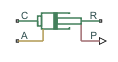# Single-Acting Hydraulic Cylinder (Simple)

(To be removed) Basic functionality of single-acting hydraulic cylinder

The Hydraulics (Isothermal) library will be removed in a future release. Use the Isothermal Liquid library instead. (since R2020a)

## Library

Hydraulic Cylinders

•## Description

The Single-Acting Hydraulic Cylinder (Simple) block represents a simplified version of a hydraulic cylinder, developed for applications where only the basic cylinder functionality must be reproduced, in exchange for better numerical efficiency. For these reasons, such factors as fluid compressibility, friction, and leakages are assumed to be negligible. The hard stops are assumed to be fully inelastic, to eliminate any possible oscillations at the end of the stroke. The model is especially suitable for real-time and HIL (hardware-in-the-loop) simulation, if such simplifications are acceptable.

The model is described with the following equations:

`$F=A\cdot p-{F}_{c}$`
`$q=A\cdot v$`
`$\frac{dx}{dt}=v$`
`$v={v}_{R}-{v}_{C}$`

`${x}_{E}=S-{x}_{0}$`
`${x}_{R}=-{x}_{0}$`

where

 F Force developed by the cylinder v Cylinder rod velocity vR,vC Absolute velocities of cylinder rod and cylinder case, respectively A Piston area p Pressure at the cylinder inlet q Flow rate at the cylinder inlet x Piston position x0 Initial distance between piston and the cap Fc Hard stop force xE Distance the piston can travel to fully extend from initial position xR Distance the piston can travel to fully retract from initial position Kp Penetration coefficient S Piston stroke

The main difference between this block and the Single-Acting Hydraulic Cylinder block is the representation of the hard stop:

• The Single-Acting Hydraulic Cylinder block utilizes the elastic model of the stop, which is essentially a combination of a spring and a damper, connected in parallel. The stiffness of the spring, which represents contact stiffness of colliding bodies, is very high, resulting in high-frequency, low-magnitude oscillations at the moment the bodies collide. These oscillations can hardly be noticed experimentally, but they are difficult to process by a numerical simulation and generally decrease numerical efficiency.

• The hard stop in the Single-Acting Hydraulic Cylinder (Simple) block is represented with the inelastic model, which is essentially a viscous damper with the penetration-dependent damping coefficient. This coefficient is referred to as the penetration coefficient. With inelastic model, no oscillation is generated during an impact, resulting in improved numerical robustness and efficiency. But inelastic stop model has a feature that you must consider while selecting the model: colliding bodies continue slowly moving into each other as long as the contact is loaded with the compressing force. In real life, this phenomenon is similar to collision of two bodies separated by a sizable layer of viscous liquid. It takes some time to squeeze the liquid before bodies themselves come into contact.

Connections R and C are mechanical translational conserving ports corresponding to the cylinder rod and cylinder clamping structure, respectively. Connection A is a hydraulic conserving port associated with the cylinder inlet. The physical signal output port P shows current rod extension.

Pressure at port A generates force in the direction specified by the Cylinder orientation parameter.

## Basic Assumptions and Limitations

• Friction between moving parts is not taken into account.

• Inertia effects are not taken into account.

• Fluid compressibility is not taken into account.

• Leakage flows are assumed to be negligible.

• The hard stops are assumed to be fully inelastic, as explained above.

## Parameters

Piston area

Effective piston area. The default value is `5e-4` m^2.

Piston stroke

Piston maximum travel between caps. The default value is `0.1` m.

Initial distance between piston and cap

The distance between the piston and cap at the beginning of simulation. This value cannot exceed the piston stroke. The default value is `0`, which corresponds to the fully retracted position.

Penetration coefficient

Specifies the penetration property of colliding bodies. The higher the value of the coefficient, the less the bodies penetrate into each other, but the sharper the increase of the impact force. To find out the value of the coefficient for a particular application, it is recommended to run the same model with different hard stop models (or with different cylinder models) until they show close results. The default value of the coefficient is `1e12` N/m/(m/s).

Cylinder orientation

Specifies cylinder orientation with respect to the globally assigned positive direction. The cylinder can be installed in two different ways, depending upon whether it exerts force in the positive or in the negative direction when pressure is applied at its inlet. If pressure applied at port A exerts force in negative direction, set the parameter to ```Acts in negative direction```. The default value is ```Acts in positive direction```.

## Ports

The block has the following ports:

`A`

Hydraulic conserving port associated with the cylinder inlet.

`R`

Mechanical translational conserving port associated with the cylinder rod.

`C`

Mechanical translational conserving port associated with the cylinder clamping structure.

`P`

Physical signal output port that outputs rod extension.

## Version History

Introduced in R2010a

expand all